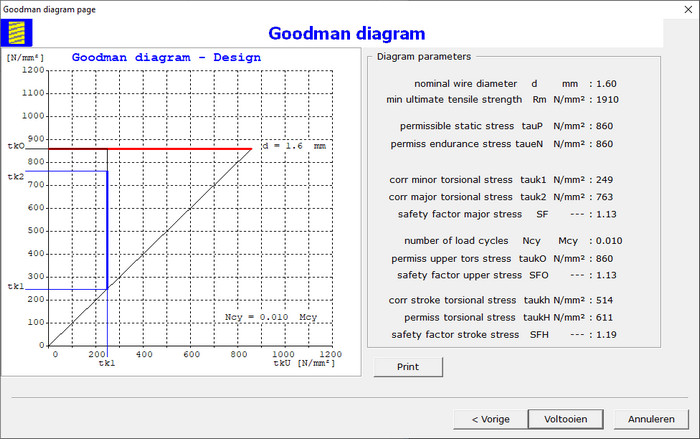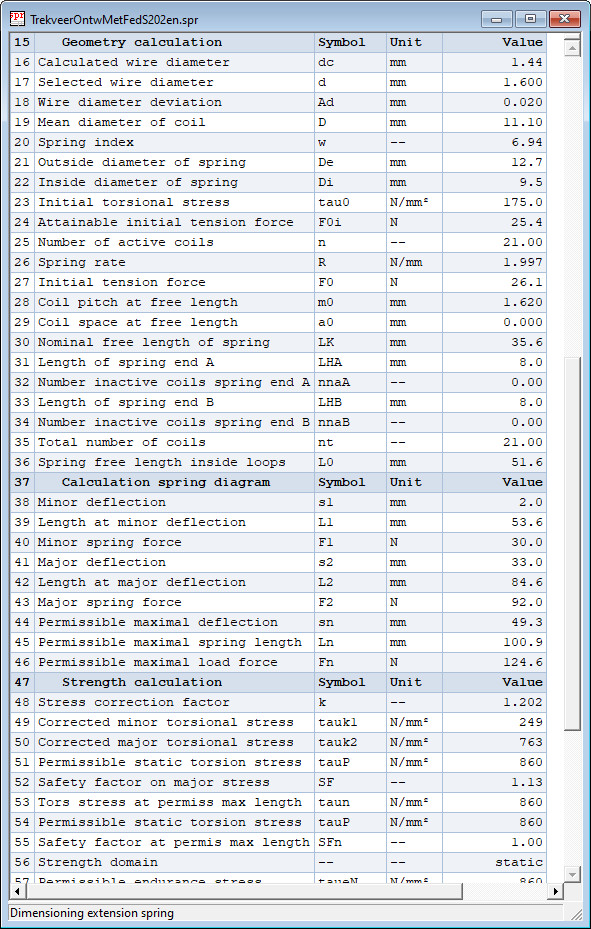### Goodman Diagram

Displayed is wizard page:The Goodman diagram is determined for the given number of load cycles and the nominal wire diameter. The safety factors for static and dynamic loading are calculated. The diagram is printed with a mouse click on the command button Print.

### Design Calculation results

The design calculation results are displayed in table form on the screen, as in following example:Click here on Calculation Report Design Extension Spring to see a complete calculation report for design of an extension spring in a target blank page.

## Verification Calculation of Extension Spring

Verification calculation according standard DIN EN 13906-2. The verification calculation can only be done if the dimensions of the spring and the load are known. The safety factors on failure are determined for static and dynamic loading. Same calculation steps are peformed as in the design calculation, except those for calculation of the dimensions. The natural frequency of the coils in longitudinal direction is calculated.

Click here on Calculation Report Verification Extension Spring to see a complete calculation report for verification extension spring in a target blank page.

Go to next web page for torsion spring calculation.